# A Free Unit Converter App for Engineers

June 17, 2019

In the engineering design and calculation practice, various unit systems will be encountered. It is very important to convert these engineering quantities accurately and quickly. WELSIM recently released a free unit converter application — UnitConverter. This application helps engineers convert quantity values between units correctly and quickly.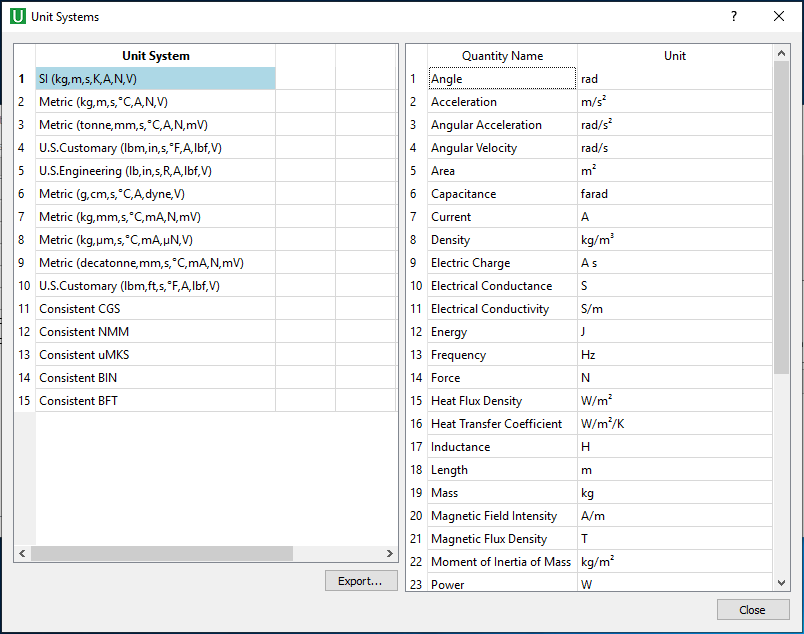Currently, this application supports 15 default unit systems, including:

SI (kg, m, s, K, A, N, V) MKS Standard (kg, m, s, C, A, N, V) NMMTON Standard (t, mm, s, C, A, N, mV) BIN Standard (lbm,in,s,F,A,lbf,V) US Engineering (lb,in,s,R,A,lbf,V) CGS Standard (g, cm, s, C, A, dyne, V) NMM Standard (kg, mm, s, C, mA, N, mV) UMKS Standard (kg, um, s, C, mA, uN, V) NMMDAT Standard (dt, mm, s, C, mA, N, mV) BFT Standard (lbm, ft, s, F, A, lbf, V) CGS Consistent (g, m, s, C, A, dyne, V) NMM Consistent (t,m,s,mC,mA,tmm/s2,mV) UMKS Consistent (kg, m, s, pC, pA, uN, pV) BIN Consistent (slinch,in,s,C,A,slinchin/s2,V) BFT Consistent (slug, ft, s, C, A, slug*ft/s2, V)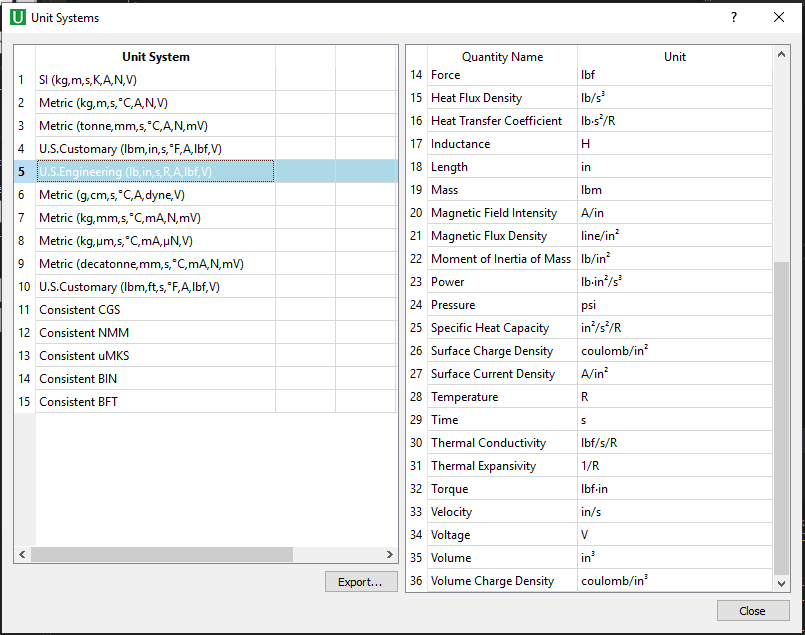Due to a large number of unit quantities, UnitConverter groups all units into six categories: Basic, Common, Mechanical, Thermal, Electrical, and Magnetic. The supported units for each category are described below.

The Base units support Angle, Current, Length, Mass, Temperature, and Time.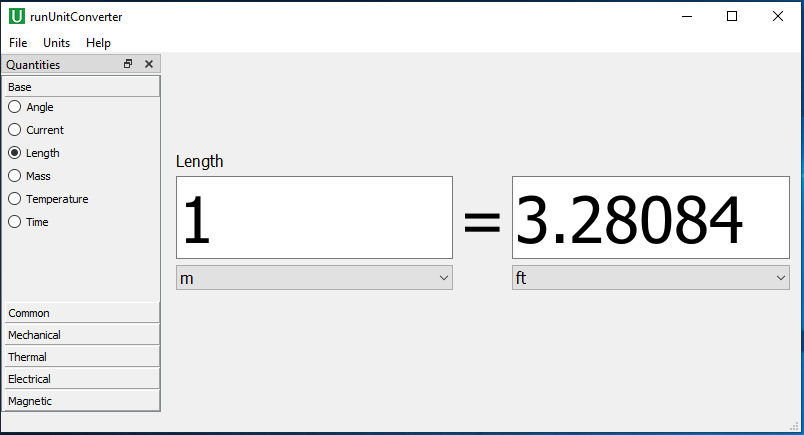The Common units support Area, Density, Energy, Frequency, Volume.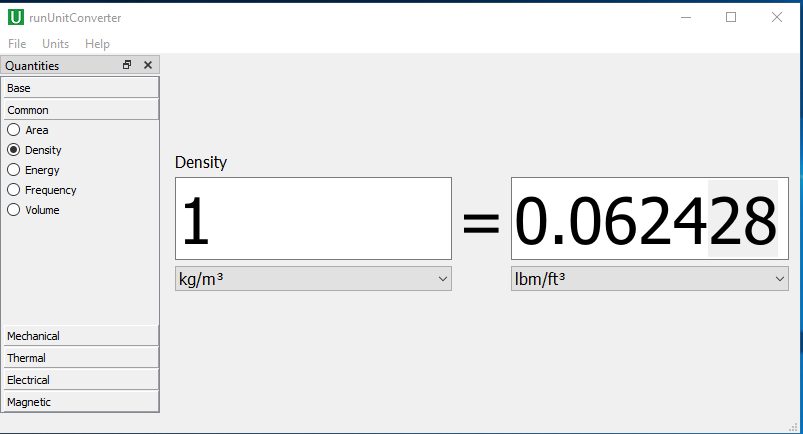The mechanical units support: Acceleration, Angular Acceleration, Angular Velocity, Force, Moment of Inertia, Power, Pressure, Torque, Velocity.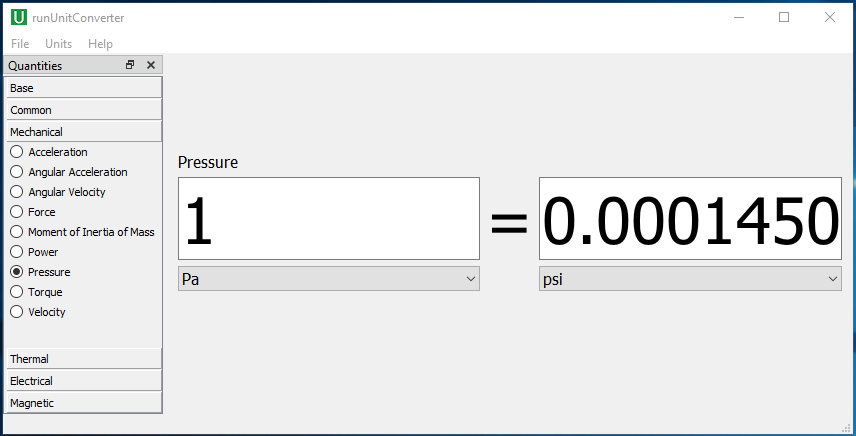The thermal units support Heat Flux Density, Heat Transfer Coefficient, Specific Heat Capacity, Thermal Conductivity, and Thermal Expansivity.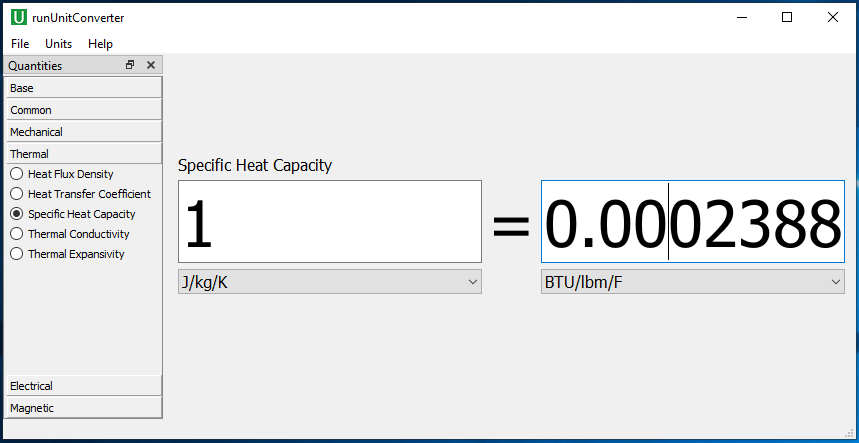The electrical units support Capacitance, Electric Charge, Electrical Conductance, Electrical Conductivity, Inductance, Surface Charge Density, Surface Current Density, Voltage, Volume Charge Density.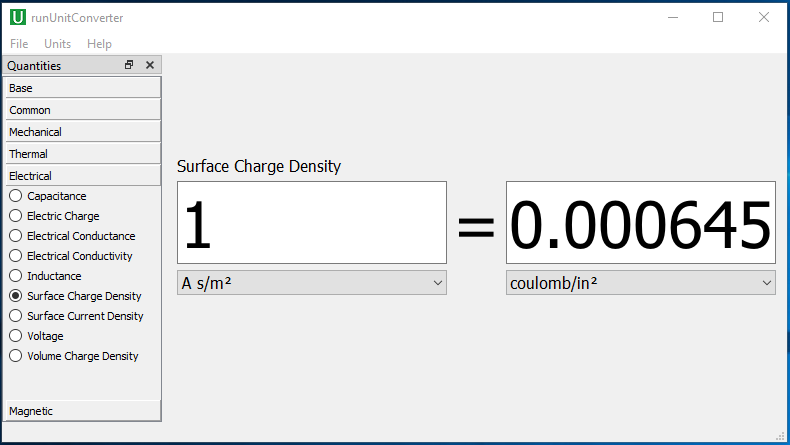The magnetic units support magnetic field strength, magnetic flux density.The current first release version already supports 15 unit systems and 36 unit quantities. The following versions will also support more unit quantities.

Click on the About option in the menu to display the information of the UnitConverter application, as well as local hardware information.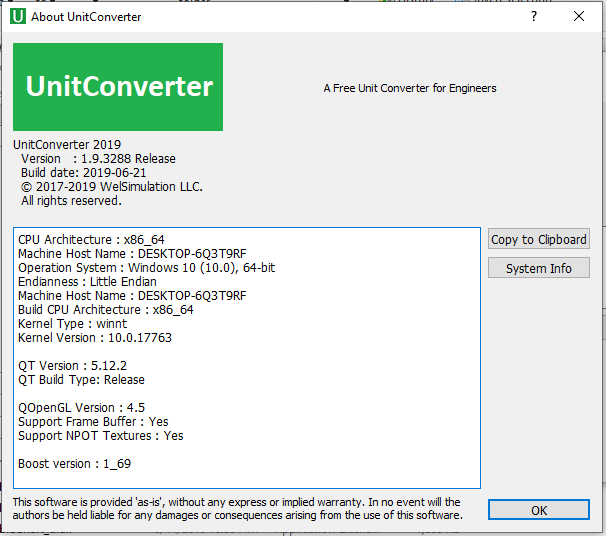Finally, this UnitConverter is free and you can download it directly from https://welsim.com/unitconverter.